## Example Questions

### Example Question #1 : How To Find The Area Of A Right Triangle

Quantitative Comparison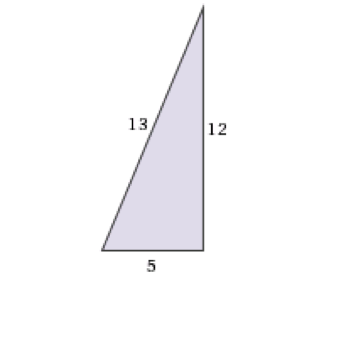Column A

Area

Column B

Perimeter




Column A and B are equal

Column A is greater

Column B is greater

Cannot be determined

Column A and B are equal

Explanation:

To find the perimeter, add up the sides, here 5 + 12 + 13 = 30. To find the area, multiply the two legs together and divide by 2, here (5 * 12)/2 = 30.

### Example Question #91 : Geometry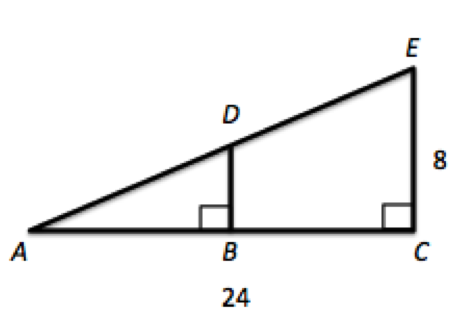Given triangle ACE where B is the midpoint of AC, what is the area of triangle ABD?

96

24

48

72

24

Explanation:

If B is a midpoint of AC, then we know AB is 12. Moreover, triangles ACE and ABD share angle DAB and have right angles which makes them similar triangles. Thus, their sides will all be proportional, and BD is 4. 1/2bh gives us 1/* 12 * 4, or 24.

### Example Question #44 : Triangles

What is the area of a right triangle with hypotenuse of 13 and base of 12?

25

30

156

78

60

30

Explanation:

Area = 1/2(base)(height). You could use Pythagorean theorem to find the height or, if you know the special right triangles, recognize the 5-12-13. The area = 1/2(12)(5) = 30.

### Example Question #1 : Right Triangles

Quantitative Comparison

Quantity A: the area of a right triangle with sides 10, 24, 26

Quantity B: twice the area of a right triangle with sides 5, 12, 13

The relationship cannot be determined from the information given.

The two quantities are equal.

Quantity B is greater.

Quantity A is greater.

Quantity A is greater.

Explanation:

Quantity A: area = base * height / 2 = 10 * 24 / 2 = 120

Quantity B: 2 * area = 2 * base * height / 2 = base * height = 5 * 12 = 60

Therefore Quantity A is greater.

### Example Question #2 : Right Triangles

Quantitative Comparison

Quantity A: The area of a triangle with a height of 6 and a base of 7

Quantity B: Half the area of a trapezoid with a height of 6, a base of 6, and another base of 10

Quantity B is greater.

The two quantities are equal.

Quantity A is greater.

The relationship cannot be determined from the information given.

Quantity B is greater.

Explanation:

Quantity A: Area = 1/2 * b * h = 1/2 * 6 * 7 = 42/2 = 21

Quantity B: Area = 1/2 * (b1 + b2) * h = 1/2 * (6 + 10) * 6 = 48

Half of the area = 48/2 = 24

Quantity B is greater.

### Example Question #47 : Triangles

The radius of the circle is 2. What is the area of the shaded equilateral triangle?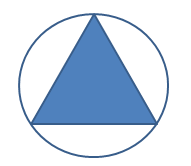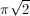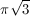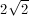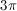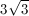Explanation:

This is easier to see when the triangle is divided into six parts (blue). Each one contains an angle which is half of 120 degrees and contains a 90 degree angle. This means each triangle is a 30/60/90 triangle with its long side equal to the radius of the circle. Knowing that means that the height of each triangle is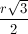and the base is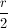.

Applying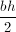and multiplying by 6 gives).Tired of practice problems?

Try live online GRE Math prep today.